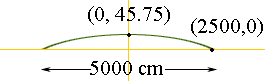Quandaries and Queries Francis Have you ever walked on a football field covered with artificial turf? If so, you probably noticed that the field is not flat. The profile of the surface is arched and highest in the centre, permitting rainwater to drain away quickly. height from base to highest point- 45.75 centimetres distance of the field- 50 metres a) The diagram shows the profile of an actual field, viewed from the end of the field. Assuming that the cross-section is a parabola, find the algebraic model that describes this shape. b) Use your equation to determine the distance from the sidelines where the field surface is 20 cm above the base line. Hi Francis, You didn't send us a diagram so I guessed at the diagram below. I changed all the dimesnions to centimeters.I put a coordinate system on the diagram (the axes are in yellow). Since the shape of the cross-section is a parabola, and in my diagram the parabola has its axis on the Y-axis and opens downward, the equation of the parabola is y = -a x2 + b where a is positive. The points (0, 45.75) and (2500, 0) are on the parabola so they satisify the equation. Substitute the two points into the equation and you will get two equations in a and b. Solve these equations to find a and b and then you will have the equation of the parabola. Harley Go to Math Central